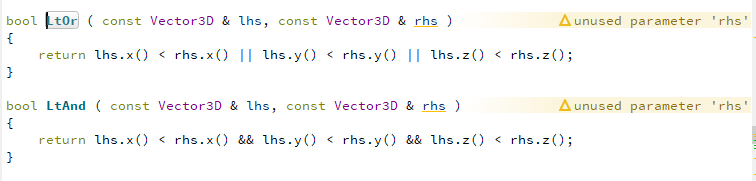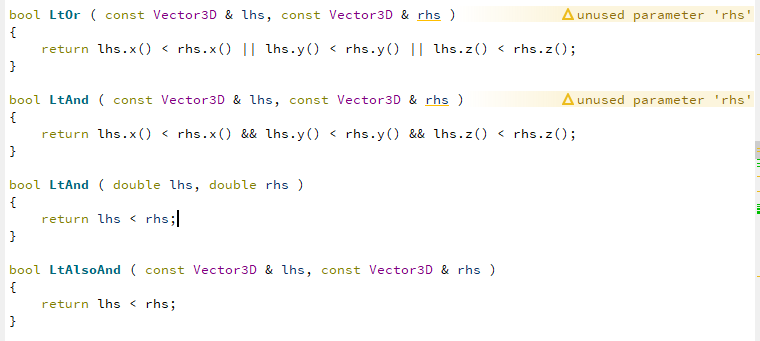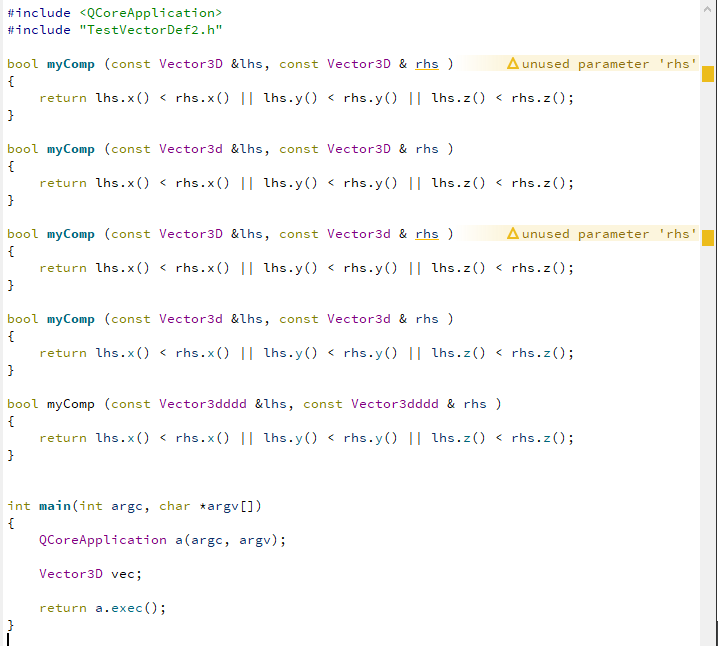# Funny bug in clang model indication in creator 4.7.0

• Just stumpled over this with newest Qt creator 4.7.0I wonder if this is another problem by using an older Qt lib version Qt5.4.2 MinGW 32 bit pre-built.
OS is windows 10 64 bit

• Caused by member functions• @koahnig

Thanks for the report. But we need a minimal example to reproduce it... otherwise its hard to reproduce.

Thanks

• First cases were observed with template which inherits from another template. However, with some additional playing around I can limit the problem and represent by:

TestClangIssue.pro

``````QT -= gui

CONFIG += c++11 console
CONFIG -= app_bundle

# The following define makes your compiler emit warnings if you use
# any feature of Qt which as been marked deprecated (the exact warnings
# deprecated API in order to know how to port your code away from it.
DEFINES += QT_DEPRECATED_WARNINGS

# You can also make your code fail to compile if you use deprecated APIs.
# In order to do so, uncomment the following line.
# You can also select to disable deprecated APIs only up to a certain version of Qt.
#DEFINES += QT_DISABLE_DEPRECATED_BEFORE=0x060000    # disables all the APIs deprecated before Qt 6.0.0

SOURCES += \
main.cpp

# Default rules for deployment.
qnx: target.path = /tmp/\$\${TARGET}/bin
else: unix:!android: target.path = /opt/\$\${TARGET}/bin
!isEmpty(target.path): INSTALLS += target

#    TestVectorDef.h \
TestVectorDef2.h
``````

TestVectorDef2.h:

``````#ifndef TESTVECTORDEF_H
#define TESTVECTORDEF_H

template <class T>
class Vector3T // : public VectorNT <T, 3>
{
T Data;
public:

//  constructors
//! default constructor
Vector3T ();
//! constructor initializes all three components with the supplied value
Vector3T (T);
//! constructor initializes with the three supplied values
Vector3T (T, T, T);

//  components
T x () const;
T y () const;
T z () const;
};
template <class T>
inline Vector3T <T> :: Vector3T ()
{
}
template <class T>
inline Vector3T <T> :: Vector3T (T val)
{
Data = val;
Data = val;
Data = val;
}
template <class T>
inline Vector3T <T> :: Vector3T (T x, T y, T z)
{
Data  = x;
Data  = y;
Data  = z;
}
template <class T>
inline T Vector3T <T> :: x () const
{
return Data ;
}
template <class T>
inline T Vector3T <T> :: y () const
{
return Data ;
}
template <class T>
inline T Vector3T <T> :: z () const
{
return Data ;
}

typedef Vector3T <double> Vector3D;

class Vector3d
{
double Data;
public:

//  constructors
//! default constructor
Vector3d ();
//! constructor initializes all three components with the supplied value
Vector3d (double );
//! constructor initializes with the three supplied values
Vector3d (double, double,double);

//  components
double x () const;
double y () const;
double z () const;
};
inline Vector3d:: Vector3d ()
{
}
inline Vector3d :: Vector3d (double val)
{
Data = val;
Data = val;
Data = val;
}
inline Vector3d :: Vector3d (double x, double y, double z)
{
Data  = x;
Data  = y;
Data  = z;
}
inline double Vector3d :: x () const
{
return Data ;
}
inline double Vector3d :: y () const
{
return Data ;
}
inline double Vector3d :: z () const
{
return Data ;
}
typedef Vector3d Vector3dddd;
#endif // TESTVECTORDEF_H
``````

main.cpp

``````#include <QCoreApplication>
#include "TestVectorDef2.h"

bool myComp (const Vector3D &lhs, const Vector3D & rhs )
{
return lhs.x() < rhs.x() || lhs.y() < rhs.y() || lhs.z() < rhs.z();
}

bool myComp (const Vector3d &lhs, const Vector3D & rhs )
{
return lhs.x() < rhs.x() || lhs.y() < rhs.y() || lhs.z() < rhs.z();
}

bool myComp (const Vector3D &lhs, const Vector3d & rhs )
{
return lhs.x() < rhs.x() || lhs.y() < rhs.y() || lhs.z() < rhs.z();
}

bool myComp (const Vector3d &lhs, const Vector3d & rhs )
{
return lhs.x() < rhs.x() || lhs.y() < rhs.y() || lhs.z() < rhs.z();
}

bool myComp (const Vector3dddd &lhs, const Vector3dddd & rhs )
{
return lhs.x() < rhs.x() || lhs.y() < rhs.y() || lhs.z() < rhs.z();
}

int main(int argc, char *argv[])
{
QCoreApplication a(argc, argv);

Vector3D vec;

return a.exec();
}
``````

On windows 10 64 bit with Qt 5.10.1 pre-built with MinGW 32 bit it showsAs shown the problem seems to originate from the combination of typedef and a template. However, using the template argument directly shows that possibly the template it self is the "problem".

BTW Yes, the example does not compile directly, because some representations are duplicated, but that is not the origin of the problem. Now I am going to stop fuzzing around with this ;)

• Probably some bug in the clang parsing.
Btw

``````bool myComp (const Vector3d &lhs, const Vector3d & rhs )
bool myComp (const Vector3dddd &lhs, const Vector3dddd & rhs )
``````

should give you ambiguous overload errors.

Yes, the example does not compile directly, because some representations are duplicated, but that is not the origin of the problem.

OT:
That's a very odd way of comparing vectors ... I wouldn't expect it to really work properly.

• Probably some bug in the clang parsing.
Btw

``````bool myComp (const Vector3d &lhs, const Vector3d & rhs )
bool myComp (const Vector3dddd &lhs, const Vector3dddd & rhs )
``````

should give you ambiguous overload errors.

Exactly. That was the reason of my "BTW". I was getting lazy in this heat to redo all the pasting ;)

Yes, the example does not compile directly, because some representations are duplicated, but that is not the origin of the problem.

OT:
That's a very odd way of comparing vectors ... I wouldn't expect it to really work properly.

The myComp name stems from the reducing to minimal case activity. The original naming is in the top post and it represents better what is done.

For sure the comparison for standard vectors would be really odd. However, the routines are implemented locally with a specific application where exactly this case is repeatibly required respectively sufficient. ;)